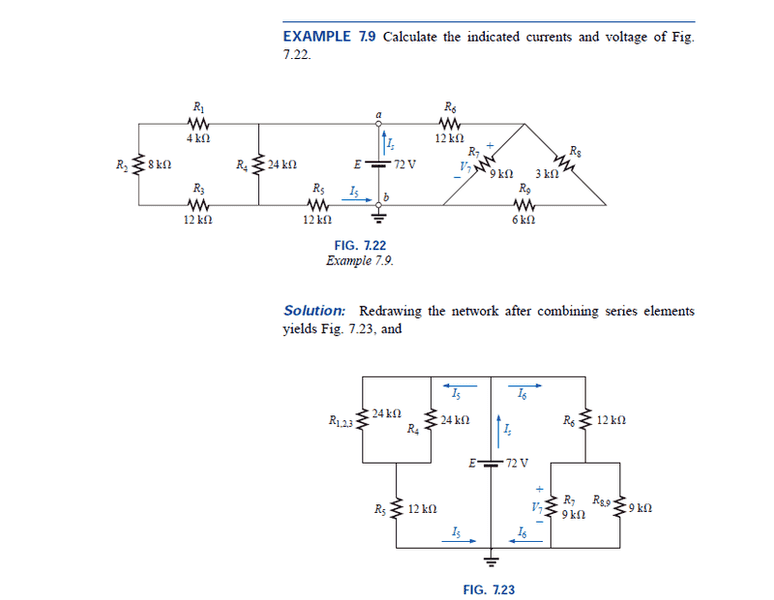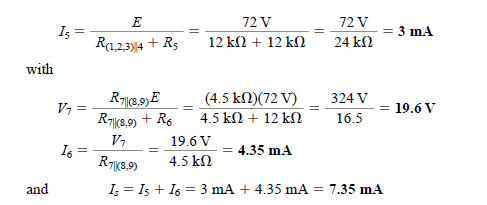# A Question in Voltage in a Circuit

• Engineering
bibo_dvd
hello guys !!

iam new at this forum and i have a questionThe quesion is : why can't i calculate V7 from Ohm's Law So V7=E/R7
and it will be equal to 72/9=8V

Please answer me and thank you guys :)

Last edited by a moderator:

## Answers and Replies

Staff Emeritus
The quesion is : why can't i calculate V7 from Ohm's Law So V7=E/R7
Hi bibo_dvd. http://img96.imageshack.us/img96/5725/red5e5etimes5e5e45e5e25.gif [Broken]

If R6 were equal to 0Ω then you could indeed say V7=E/R7

Ohms Law requires E to be the voltage across R. You can't consider a voltage from just anywhere and divide it by any resistance you choose. E must be the exact voltage across exactly that R. In your circuit, R6 is in the way, meaning that

E = (the voltage across R7) + (the voltage across R6).

Last edited by a moderator:
Gold Member
NasOxy is correct.
I think you need to back off to a simpler example and fully understand it first. Then the problem will make sense.
There is a wikipedia page for "voltage divider" you should read and understand. For the simple voltage divider (R1 in series with R2) you calculate the total current I through R1+R2 and then the voltage across R2 is I*R2.

Gold Member
Look up "Kirchoff's voltage law".....google it.

For random example, If you have one voltage source of say 100 volts and just one resistor, then and only then does the entire 100 volts goes accross that one resistor.

If you have same voltage source and say 2 resistors of equal value, then 50 volts will go accross each of them...adding up to 100 volts.

If you have same voltage source and have 2 resistors of different value say, 10 ohms and 20 ohms, then the voltage will divide across the resitors with a ratio.

100 volts X (10/(10+20)).....so you will get 33.33 volts across the 10 ohm....and 66.66 accross the 20 ohm.

To work the problem above, you simply combine the two 9 ohms in parallel and you get 4.5 ohms.
Then use voltage divider above....

72 X (4.5/(4.5+12) = 19.6 volts across that branch or 4.36 ma using V=IR which agrees with answer above.
Now that you know the 19.6 across that branch, spread the resistors out back to there originial shape....two 9 ohm resistors.

Using ohms law again, you can now check the currents again. The two branches should add up to the 4.36 ma.
19.6/ 9 ohms = 2.18 ma a piece....yes, checks out according to Kirchoffs Current law (also look up in google)

Last edited: